# MapInfo Pro

View Only

## Calculate the Segment Line Direction in MapInfo in a geographic coordinate system (GCS)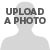#### Mike Woodbury08-26-2021 04:04#### Peter Horsbøll Møller08-27-2021 02:20#### Mike Woodbury08-27-2021 18:09#### Mike Woodbury08-30-2021 00:29#### Uffe Kousgaard08-27-2021 09:14#### Peter Horsbøll Møller09-16-2021 02:27• #### 1.  Calculate the Segment Line Direction in MapInfo in a geographic coordinate system (GCS)

Posted 08-26-2021 04:04

Hi,

Does anyone know how to calculate the line direction of a line segment in MapInfo in a geographic coordinate system (GCS) over a  projected coordinate system (PCS)

There is a function in Discover however, you need to work in a projected coordinate system (PCS) and it will not work in a geographic coordinate system (GCS)

As well, we work over the entire Australia, so we cross a number of zones e.g. MGA GDA94 z49-52 in WA alone

I have also checked out Peter H PPT however, the Calc function described works on a single line / segment (using MapCAD)

Aim:

Calc the Segment Line direction = azimuth for all of WA faults in geographic coordinate system (GCS); Long/Lat GDA94

Please assist with tools or suggestion in MapInfo, as Discover tool doesn't suite our needs and will not work with GCS

Cheers

PS. A links for QGIS to calc the line direction below:

With PyQGIS in the Python console, see How to add Direction and Distance to attribute table? for the azimuths of the segments of a line (with the azimuth functions of Points: point1.azimuth(point2))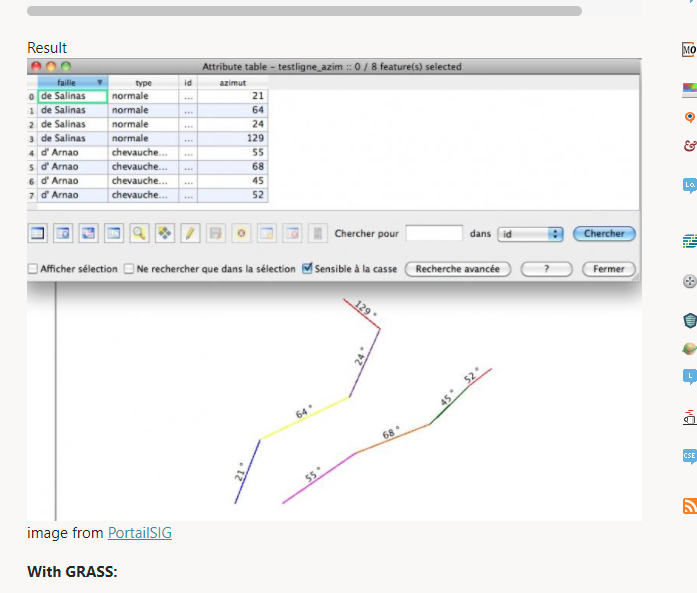------------------------------
Mike Woodbury
Knowledge Community Shared Account
------------------------------

• #### 2.  RE: Calculate the Segment Line Direction in MapInfo in a geographic coordinate system (GCS)

Posted 08-27-2021 02:20
Hi Mike

Would you be able to verify that the values from this formula are correct?
You'd have to select a single polyline (with a single line segment) and then run this script either from the MapBasic window or the SQL Window.

`Dim oSel As Object`
`Dim fLong1 As Float`
`Dim fLong2 As Float`
`Dim fLat1 As Float`
`Dim fLat2 As Float `
`Dim fLongDiff As Float`
`Dim fX As Float`
`Dim fY As Float`
`Dim fBearing As Float`

`Fetch First From Selection`
`oSel = Selection.OBJ`

`Set CoordSys Earth Projection 1, 0`
`fLong1 = ObjectNodeX(oSel, 1, 1)`
`fLat1 = ObjectNodeY(oSel, 1, 1)`
`fLong2 = ObjectNodeX(oSel, 1, ObjectInfo(oSel, OBJ_INFO_NPNTS))`
`fLat2 = ObjectNodeY(oSel, 1, ObjectInfo(oSel, OBJ_INFO_NPNTS))`

`fLongDiff = (fLong2 - fLong1)`
`Print "Long Diff.: " & FormatNumber\$(fLongDiff)`

`fY = Sin(fLongDiff) * Cos(fLat2)`
`fX = Cos(fLat1) * Sin(fLat2) - Sin(fLat1) * Cos(fLat2) * Cos(fLongDiff)`

`fBearing = Atn(fY / fX) * 57.29577951`
`Print "Bearing 1: " & FormatNumber\$(fBearing)`
`fBearing = IIf(fBearing >= 0, fBearing, fBearing + 360)`

`Print "Bearing 2: " & FormatNumber\$(fBearing)`

You can also adjust the coordinate system to be a different lat/long based.

------------------------------
Peter Horsbøll Møller
Principal Presales Consultant | Distinguished Engineer
Precisely | Trust in Data
------------------------------

• #### 3.  RE: Calculate the Segment Line Direction in MapInfo in a geographic coordinate system (GCS)

Posted 08-27-2021 18:09
Hi Peter,

You ongoing support is much appreciated.

Matt and myself (from our office)  thank you very much.

Very happy you could assist. Discover support was unable to assist.

Stay safe

Cheers Mike

Sent from Mike W iPhone

• #### 4.  RE: Calculate the Segment Line Direction in MapInfo in a geographic coordinate system (GCS)

Posted 08-30-2021 00:29

Hi Peter,

Thank you again!

We ran the script on a single selection in the mapbasic window and the outcome is below – it looks like it worked

WRT bearing, there are 2; bearing 1 and 2. I can confirm MIP is applying 000-180 to a N-S line (with North up the page '000')

Is there any way of adding to the script, and place the outcome; result / bearing (1 & 2) into the table in a field named e.g. bearing1 and 2? Table would be editable?

Also can this script be applied to a large section of lines in one go, and write the bearing 1 & 2 to the editable table?

Thanks again!

Cheers, Mike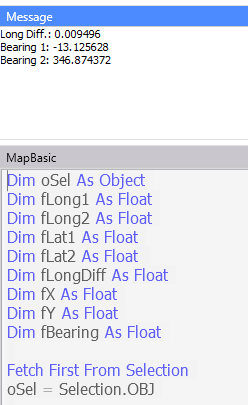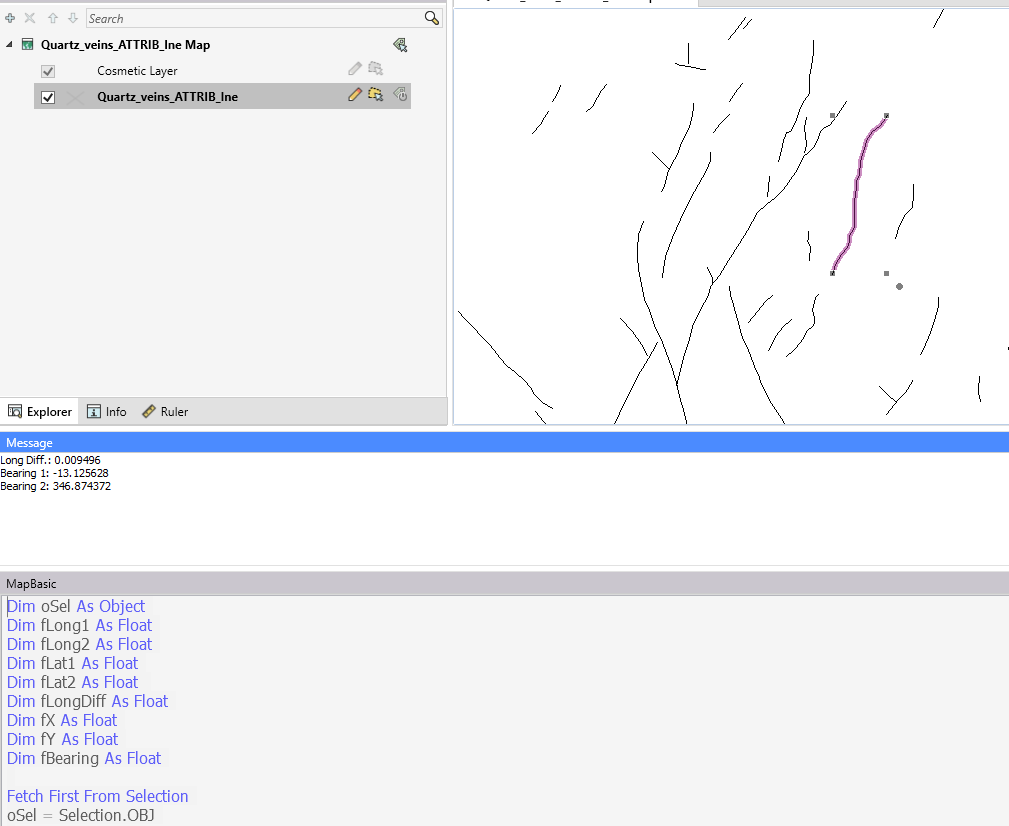• #### 5.  RE: Calculate the Segment Line Direction in MapInfo in a geographic coordinate system (GCS)

Posted 08-27-2021 09:14
What Peter suggests, is the alternative formula here:

https://edwilliams.org/avform147.htm#Crs

------------------------------
Uffe Kousgaard
ROUTEWARE
Roskilde
------------------------------

• #### 6.  RE: Calculate the Segment Line Direction in MapInfo in a geographic coordinate system (GCS)

Posted 09-16-2021 02:27
Hi Mike

Sorry, I got drawn into some other business and never got back to you.
I have now built the function into a small tool that publishes the calculation as a function inside MapInfo Pro.

You can use it via the SQL Window or MapBasic window and update a query or an entire table with one statement:
`Set CoordSys Table LINESWithBearing`
`Update LINESWithBearing `
`   Set BEARING = CalculateBearingSperical(OBJ)`

When testing the tool I did however notice that the horizontal lines seem to give the same bearing no matter if they go from west to east or from east to west. I guess my formula has an error.

Maybe someone here in the community can tell me where the mistake is:
`'Formula taken from StackExchange: `
`'https://stackoverflow.com/questions/8123049/calculate-bearing-between-two-locations-lat-long`
`'double dLon = (lng2-lng1);`
`'double y = Math.sin(dLon) * Math.cos(lat2);`
`'double x = Math.cos(lat1)*Math.sin(lat2) - Math.sin(lat1)*Math.cos(lat2)*Math.cos(dLon);`
`'double brng = Math.toDegrees((Math.atan2(y, x)));`
`'brng = (360 - ((brng + 360) % 360));`

`fLong1 = ObjectNodeX(oSel, 1, 1)`
`fLat1 = ObjectNodeY(oSel, 1, 1)`
`fLong2 = ObjectNodeX(oSel, 1, ObjectInfo(oSel, OBJ_INFO_NPNTS))`
`fLat2 = ObjectNodeY(oSel, 1, ObjectInfo(oSel, OBJ_INFO_NPNTS))`

`fLongDiff = (fLong2 - fLong1)`
`' Print "Long Diff.: " & FormatNumber\$(fLongDiff)`

`fY = Sin(fLongDiff) * Cos(fLat2)`
`fX = Cos(fLat1) * Sin(fLat2) - Sin(fLat1) * Cos(fLat2) * Cos(fLongDiff)`

`fBearing = Atn(fY / fX) * RAD_2_DEG '57.29577951`
`' Print "Bearing 1: " & FormatNumber\$(fBearing)`
`fBearing = IIf(fBearing >= 0, fBearing, fBearing + 360)`
`' Print "Bearing 2: " & FormatNumber\$(fBearing)`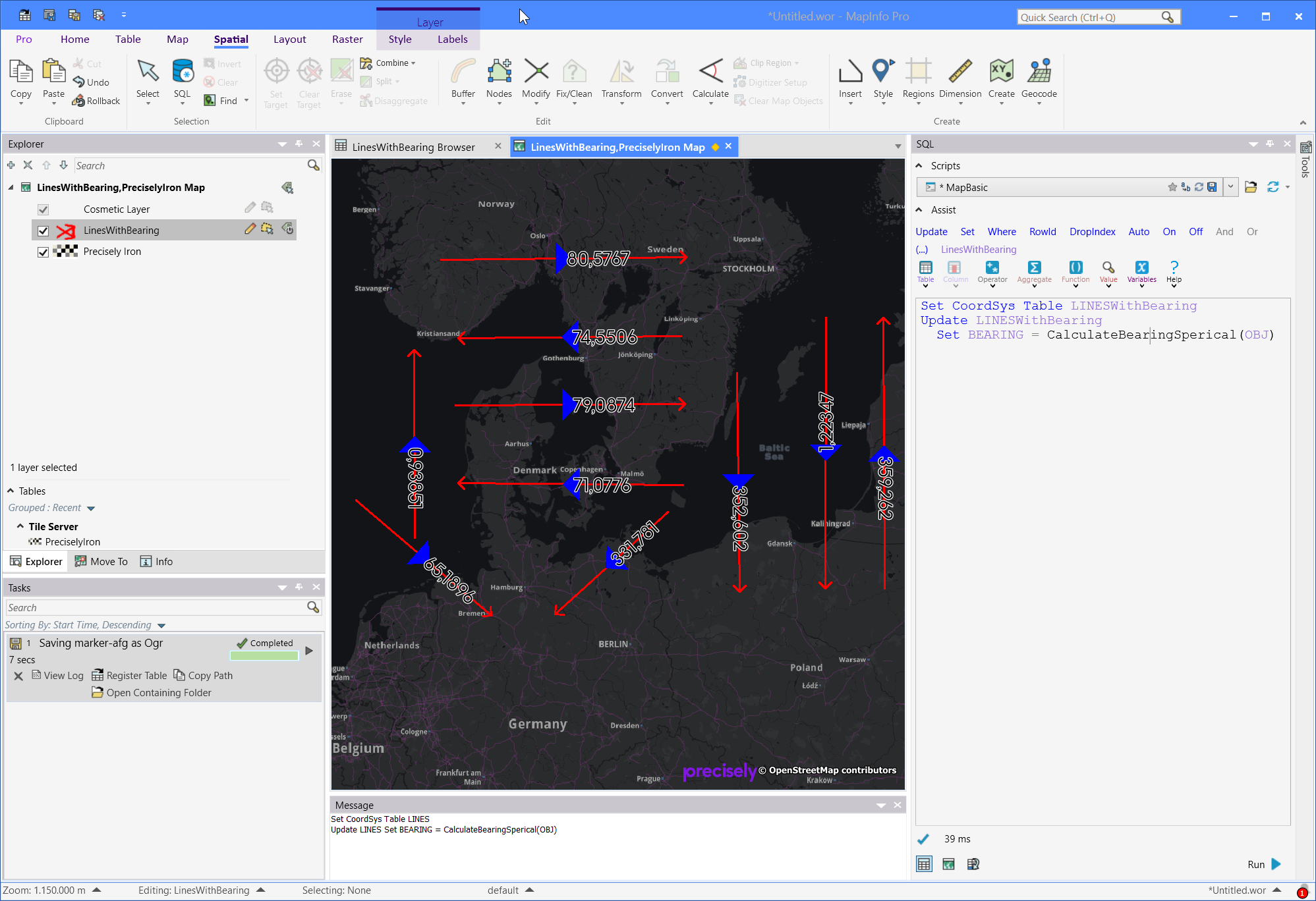I have attached the application and the MapBasic sourcecode

------------------------------
Peter Horsbøll Møller
Principal Presales Consultant | Distinguished Engineer
Precisely | Trust in Data
------------------------------

Attachment(s)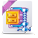• #### 7.  RE: Calculate the Segment Line Direction in MapInfo in a geographic coordinate system (GCS)

Posted 09-16-2021 02:39

Hi Peter,

Thank you, and really appreciated. We will run this afternoon, and let you know how it goes

The task was to remove N-S and E-W map boundary lines from GSWA, and other maps we work with.

Cheers, Mike